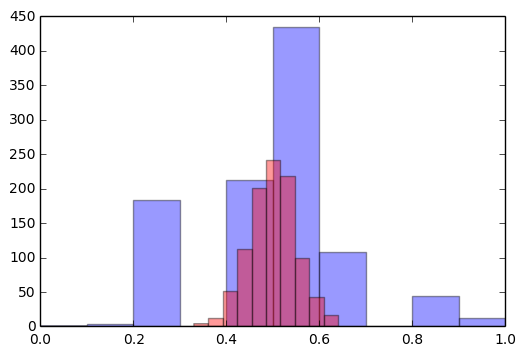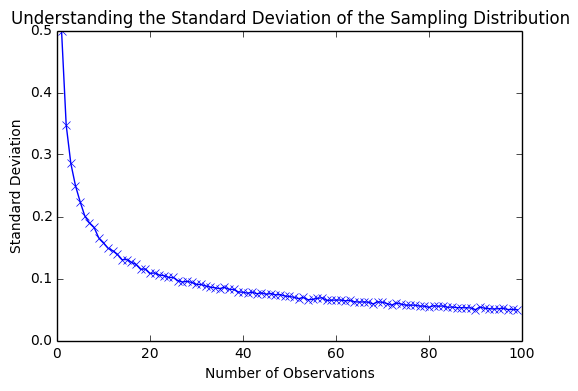# Standard Error

by Matt Johnson - Thu 16 February 2017
Tags:

Good data science involves not only making predictions from data, but also calculating confidence. Consider a binary random variable described by a Bernoulli process: $\text{P}(X \, | \, \theta) = \theta^X(1 - \theta)^{1-X}$. Furthermore, suppose we know the parameter value: $\theta = 0.5$. Now, supposing we only have a small data sample, how close is our sample mean to the population mean?

When the sample size varies, our sample means vary. We can think of this sample mean as being draw from a distribution. In fact, this distribution is known as the sampling distribution. And since it's a distribution, it will a mean and standard deviation. Let's run two experiments. For experiment A, we'll take 1000 samples (10 observations each) and compute the mean of each sample. A histogram will be used to plot these means. For experiment B, the only difference is that the samples are 100 observations each.

In :
import numpy as np
from matplotlib import pyplot as plt
%matplotlib inline
plt.xlim([0.,1.])

# Experiment 1
sample_means = [np.mean(np.random.binomial(n=1, p=0.5, size=10)) for i in xrange(1000)]
print 'Standard Deviation from Experiment 1 (n=10): {0}'.format(np.std(sample_means))
plt.hist(sample_means, alpha=0.4, color='blue')

# Experiment 1
sample_means = [np.mean(np.random.binomial(n=1, p=0.5, size=100)) for i in xrange(1000)]
print 'Standard Deviation from Experiment 2 (n=100): {0}'.format(np.std(sample_means))
plt.hist(sample_means, alpha=0.4, color='red')

plt.show()

Standard Deviation from Experiment 1 (n=10): 0.157641492
Standard Deviation from Experiment 2 (n=100): 0.0487335777468Note how both experiments are centered around 0.5 (the popoluation mean), but the widths (i.e., standard deviation) of the distributions differ. The standard deviation of a sampling distribution has a name: the standard error. Also note, that the standard deviations shrink as our sample size increase. In fact, if we didn't know theory, we could plot this relationship:

In :
n_ = range(1,100)
stds = []
for n in n_:
std = np.std([np.mean(np.random.binomial(n=1, p=0.5, size=n)) for i in xrange(1000)])
stds.append(std)

plt.title('Understanding the Standard Deviation of the Sampling Distribution')
plt.xlabel('Number of Observations')
plt.ylabel('Standard Deviation')
plt.plot(n_, stds, marker='x')
plt.show()The relationship seems to have a relationship like $\sigma \propto {n^{-\beta}}$. Where $n$ is the number of observations, and $\beta$ is some unknown positive constant. We can run linear regression after transforming into log space:

In :
from scipy import stats
slope, intercept, r_value, p_value, std_err = stats.linregress(np.log(n_), np.log(stds))
print slope, intercept, r_value, p_value, std_err

-0.499820511108 -0.695415827401 -0.999240601864 1.59399063942e-138 0.00197890720535


Now, to get the multiplicative constant to make the proportion exact, we take the exponential of the intercept:

In :
np.exp(intercept)

Out:
0.49886696229650562

The slope suggests that $\beta = \frac{1}{2}$. Thus, it looks like $\sigma_{err} = \frac{0.5}{\sqrt{n}}$ But also note, that the standard deviation of the Bernoulli distribution is equal to $\sqrt{p(1-p)}$. Since $p=0.5$, this yields $0.5$. Thus, might it be that final form is $\sigma_{err} = \frac{\sigma}{\sqrt{n}}$?

Well, let's take a step back and think. Let's consider a sample of $n$ observations.

$X_1, X_2, ..., X_n$

And compute it's sample mean

$s = \frac{1}{n} \sum_i X_i$

Now, let's compute the mean of this sample mean (i.e., the mean of the sampling distribution)

$E[s] = E[\frac{1}{n} \sum_i X_i] = \frac{1}{n} E[ \sum_i X_i] = \frac{1}{n} n \mu = \mu$

That is, the expectation value of the sampling distribution of the mean is the population mean. Let's calculate the variance:

$Var[s] = Var[\frac{1}{n} \sum_i X_i] = \frac{1}{n^2} Var[ \sum_i X_i] = \frac{1}{n^2} n \sigma^2 = \frac{\sigma^2}{n}$

And of course, the standard deviation is the square root of the variance. Therefore, the standard error is predicted to be $\frac{\sigma}{\sqrt{n}}$. This is exactly what we observed in our simulation.